Courses
Courses for Kids
Free study material
Free LIVE classes
More

# Hybridisation - JEE Important Topic## A Brief Introduction to Hybridisation

Last updated date: 22nd Mar 2023
Total views: 66.9k
Views today: 1.45k

In Chemistry, hybridisation is the combination of two or more atomic orbitals with different energies to form a new atomic orbital with a lower energy. The term "hybridisation" was first used in 1898 by British chemist Frederick Crick.

The concept of hybridisation explains the bonding properties of molecules and atoms. It is important in determining the shapes of molecules and their reactivity. In organic chemistry, hybridisation is also used to describe the sharing of electrons between atoms in a covalent bond. So read on to learn more about this fascinating concept.

## What is Hybridisation?

Hybridisation of atoms refers to the process of combining two atoms together to form a new molecule. Hybridisation can occur spontaneously or as part of a chemical reaction.

Hybridisation involves taking two atoms from different elements and combining them to create a new substance. This process can happen spontaneously or be catalysed by another agent, such as heat or light.

## Important Features of Hybridisation

• Hybridisation is a process of mixing two or more atomic orbitals with each other to form new hybrid orbitals.

• The number of hybrid orbitals formed is equal to the number of atomic orbitals involved in the process.

• The energy of these new hybrid orbitals is lower than that of the original atomic orbitals. This results in a decrease in overall energy for the atom, making it more stable.

• The shapes of molecules can be predicted by looking at the types of orbitals involved in their formation. For example, if two sp orbitals form a molecule, it will be linear in shape (like $C{{O}_{2}}$).

## Types of Hybridisation

The term 'hybridisation' refers to the process of combining two or more atomic orbitals into new orbitals with different shapes and energies. The resulting orbitals are called 'hybrids'. One type of hybridisation that can occur is called 'diagonal hybridisation'. This occurs when two orbitals on the same atom are combined to form a single hybrid orbital. The most common example of this is when two p-orbitals combine to form a single sp-hybrid orbital.

The resulting hybrids have properties that are intermediate between those of the original orbitals. For example, an sp-hybridised orbital has one lobe that is larger than the other, and its energy is somewhere between that of an s-orbital and a p-orbital.

There are three types of hybridisation: sp3, sp2 and sp. The type of hybridisation depends on the number of valence orbitals being combined.

• sp: This involves the combination of two orbitals - one s orbital and one p orbital. The resulting molecular orbital has 50% s character and 50% p character. Diagonal hybridisation of atom is present in acetylene (${{C}_{2}}{{H}_{2}}$).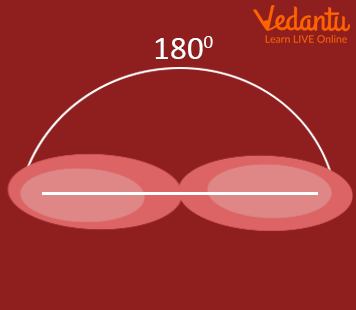SP Hybridisation

• sp2 : This type involves the combination of three orbitals - one s orbital and two p orbitals. The resulting molecular orbital has 33% s character and 67% p character. Examples include ethene (${{C}_{2}}{{H}_{4}}$) and propene ($C{}_{3}{{H}_{6}}$).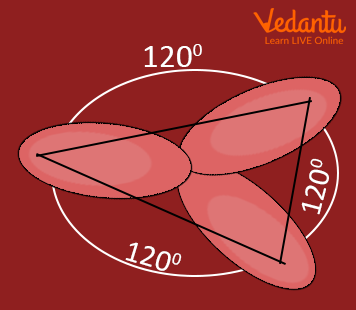$s{{p}^{2}}$ Hybridisation

• sp3 : This type of hybridization occurs when four orbitals are involved in bonding - one s orbital and three p orbitals. The resulting molecular orbital has 25% s character and 75% p character. Examples include methane ($C{{H}_{4}}$) and ethane (${{C}_{2}}{{H}_{6}}$).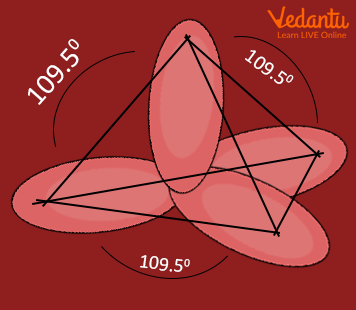$s{{p}^{3}}$ Hybridisation

• sp3d : This type of hybridisation occurs when four orbitals are involved in bonding - one s orbital, three p orbitals and one d orbitals. One example of sp3d is pcl5.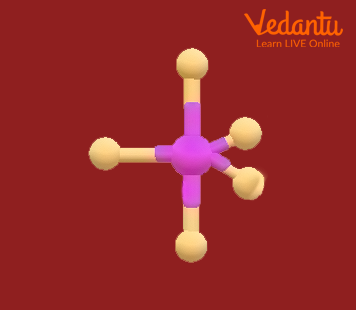$s{{p}^{3}}d$ Hybridisation

• sp3d2 : This type of hybridisation occurs when four orbitals are involved in bonding - one s orbital, three p orbitals and two d orbitals. They are inclined at an angle of 90 degrees to one another.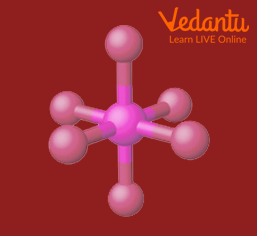sp3d2 Hybridisation

## Shapes of Hybridisation

• Linear: The sp hybridisation is caused by the interaction of two-electron groups; the orbital angle is 180°.

• Trigonal planar: Three electron groups are involved, resulting in $s{{p}^{2}}$ hybridisation; the orbitals are 120° apart.

• Tetrahedral: Four electron groups are involved, resulting in $s{{p}^{3}}$ hybridisation; the orbital angle is 109.5°.

• Trigonal bipyramidal: Five electron groups are involved, resulting in $s{{p}^{3}}d$ hybridisation; the orbital angles are 90° and 120°.

• Octahedral: Six electron groups are involved, resulting in $s{{p}^{3}}{{d}^{2}}$ hybridisation; the orbitals are 90° apart.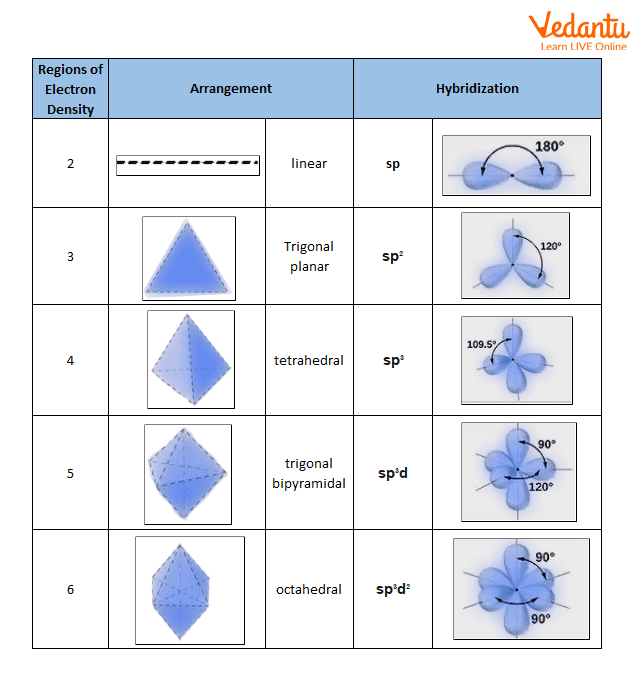Shapes of Hybridisation

## Define Hybridisation of Atom

An atom is the basic unit of an element that consists of a nucleus composed of protons and neutrons with electrons orbiting around this nucleus. The term 'hybridisation' refers to the process whereby atoms combine to form molecules by sharing electrons. This results in the formation of new atomic orbitals which are a combination of the original orbitals. The type of hybridisation that occurs depends on the number of valence electrons that each atom has.

There are various hybridisation atom examples and the most common type of hybridisation is known as sp3 hybridisation. This involves the mixing or 'hybridising' of one s orbital and three p orbitals to form four new atomic orbitals, each with a different shape. These four orbitals can then accommodate a total of eight electrons, which is the maximum number possible for this type of hybridisation. The resulting molecule has a tetrahedral structure with bond angles of 109.5 degrees.

Examples of molecules that undergo sp3 hybridisation include methane ($C{{H}_{4}}$), ethane (${{C}_{2}}{{H}_{6}}$), propane (${{C}_{3}}{{H}_{8}}$) and butane (${{C}_{4}}{{H}_{10}}$). All these molecules have a central carbon atom that is bonded to four hydrogen atoms, resulting in a stable molecule with no lone pairs. Other types of hybridisation include $s{{p}^{2}}$ and sp hybridisation.

## How to Find the Hybridisation of an Atom?

### Rules for Finding the Type of Hybridisation

To understand the type of hybridisation in a compound or an ion, the following rules must be followed:

• Calculate the total number of valence electrons.

• Calculate the number of duplex or octet OR.

• Determine the number of lone pairs of electrons.

• Number of used orbital = Number of duplex or octet + Number of lone pairs of electrons.

• If there is no lone pair of electrons then the geometry of orbitals and molecules is different.

## Summary

The hybridisation of atoms refers to the process of combining two atoms together to form a new molecule. Hybridisation can occur spontaneously or as part of a chemical reaction. It involves taking two atoms from different elements and combining them to create a new substance. The term "hybridisation" was first used in 1898 by British chemist Frederick Crick. There are three types of hybridisation: $s{{p}^{3}}$, $s{{p}^{2}}$, and sp. The type of hybridisation depends on the number of valence orbitals being combined.

## FAQs on Hybridisation - JEE Important Topic

1. What is hybridisation?

In Chemistry, hybridisation is the concept of mixing atomic orbitals into new hybrid orbitals (with different energies, shapes, etc., than the original atomic orbitals) suitable for the pairing of electrons to form chemical bonds in valence bond theory. Hybridisation is possible because the orbitals of an atom are not always symmetrical with respect to the nucleus of the atom (the centre of electron density) and thus can sometimes interact more strongly with some atoms or groups of atoms than others. Hybridisation often occurs when an atom engages in covalent bonding with another atom (or group of atoms). The resulting orbital shapes are very important in understanding many properties, such as acidity/basicity, reactivity, solubility, etc. that determine how molecules behave both individually and in reactions with each other.

2. Why do we need to study hybridisation?

We need to study hybridisation because it helps us understand molecular structure and behaviour. Many physical and chemical properties depend on the shape of molecules, which in turn depends on the arrangement of electrons around the nuclei. The way electrons are arranged in orbitals also affects things like reactivity and bond strength. By understanding how hybridisation works, we can better predict how molecules will behave in various situations.

3. What types of orbitals can be involved in hybridisation?

Generally speaking, any type of orbital can be involved in hybridisation - s-orbitals, p-orbitals, d-orbitals, and f-orbitals. The most common type of hybridisation involves sp-orbitals (a mix between an s-orbital and a p-orbital), but there are many other possibilities too.